# Multiplication Word Problems PrintableFeb 06, 2023By Printablee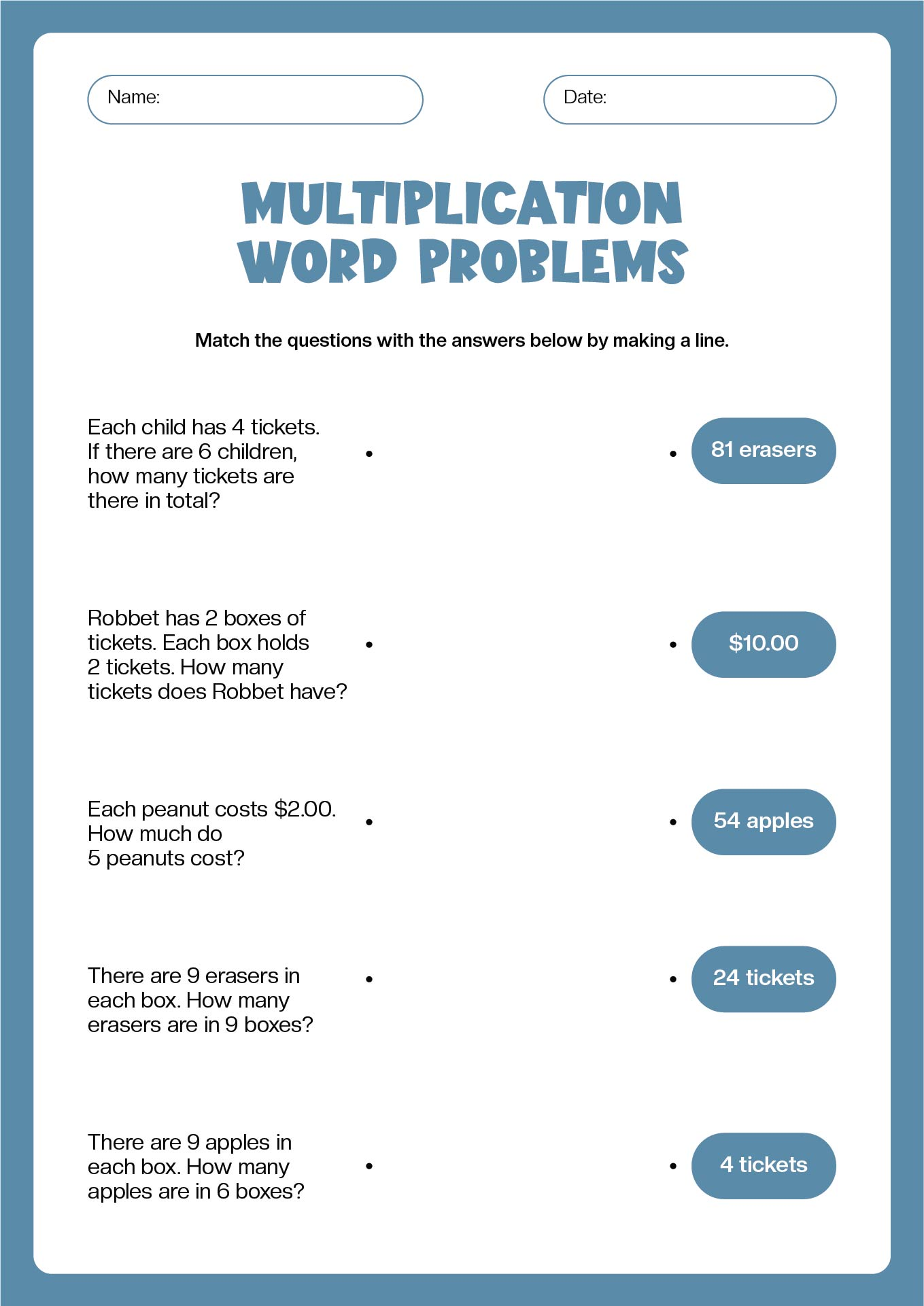Encourage your kids to complete their level upgrade in basic math using multiplication word problems. This will elevate their problem-solving skills improve from good to remarkable. These practices are a great resource for young pupils to carefully tackle a variety of well-described narrative problems.

The fundamental concept is that there are groups of objects that are the same size, and kids merely need to be able to identify them, whether they are towers, pizza slices, hats, or anything else. The lesson's word problems also include addition and subtraction, forcing students to use their reasoning skills rather than just applying the current operation (multiplication) without first comprehending the issue.

Watch your child's computing abilities grow like bunny rabbits!

## How do I identify multiplication word problems?

When there are equal groups of the same quantity, multiplication may be used to get the result. We may get the total by multiplying the quantity in each category by the number of groupings. Reading the question in its entirety and making an effort to comprehend the context is the best approach to spotting a multiplication word problem.

If you need a total and there are several groups with the same number of elements, we have a multiplication problem. Keywords may make multiplication easier to find. Several terms for multiplication include in all, altogether, total, each, and groups of. These terms are simply a hint of multiplication, so we should always look at the question's context. Words like "altogether" and "in all," for instance, may denote addition instead.

## How do you solve simple mathematical story problems?

Here are some simple steps you can deliver for kids to follow and create an answer path for their assignment:

1. Recognize the numbers in the inquiry.
2. Determine which of these figures indicates the size of each group.
3. Find which of these figures indicates the number of groupings.
4. Use long multiplication or a times table to multiply these two numbers.

These examples will focus on straightforward word problems using multiplication where the numbers being multiplied are fewer than 12 and there is only a short paragraph of text.

Try out these steps with your children. The first thing to do is introduce the whole concept in understandable language. Thereupon, you can find worksheets with interesting objects inside like fruits, blocks, animals, or anything to manipulate children so that they gain more interest in solving the problem. Your job also includes making a narrative from each question so that your children can gain more interest in solving the problems.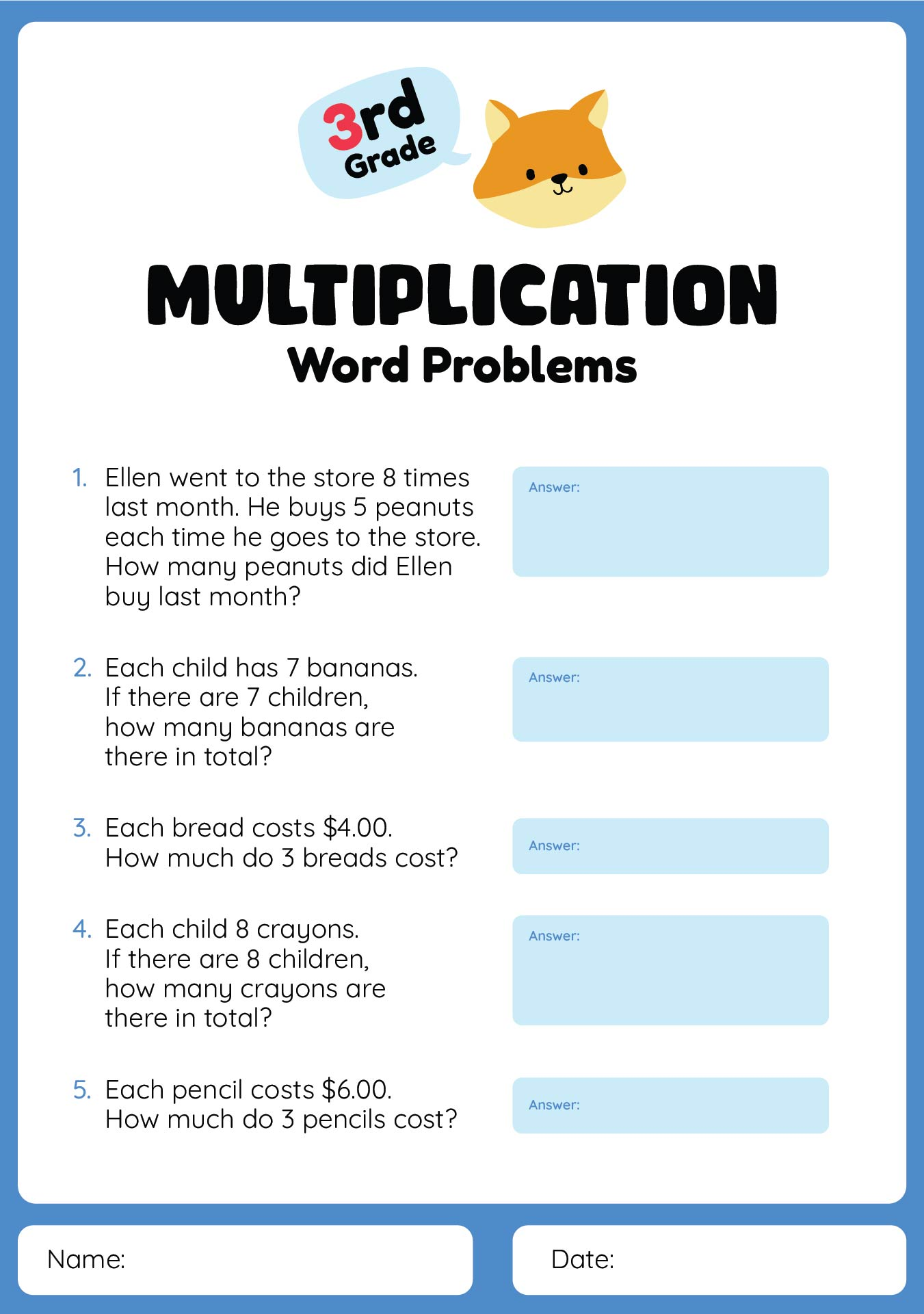We also have more printable word you may like:
First 100 Fry Words Printable
First 100 Sight Words Printable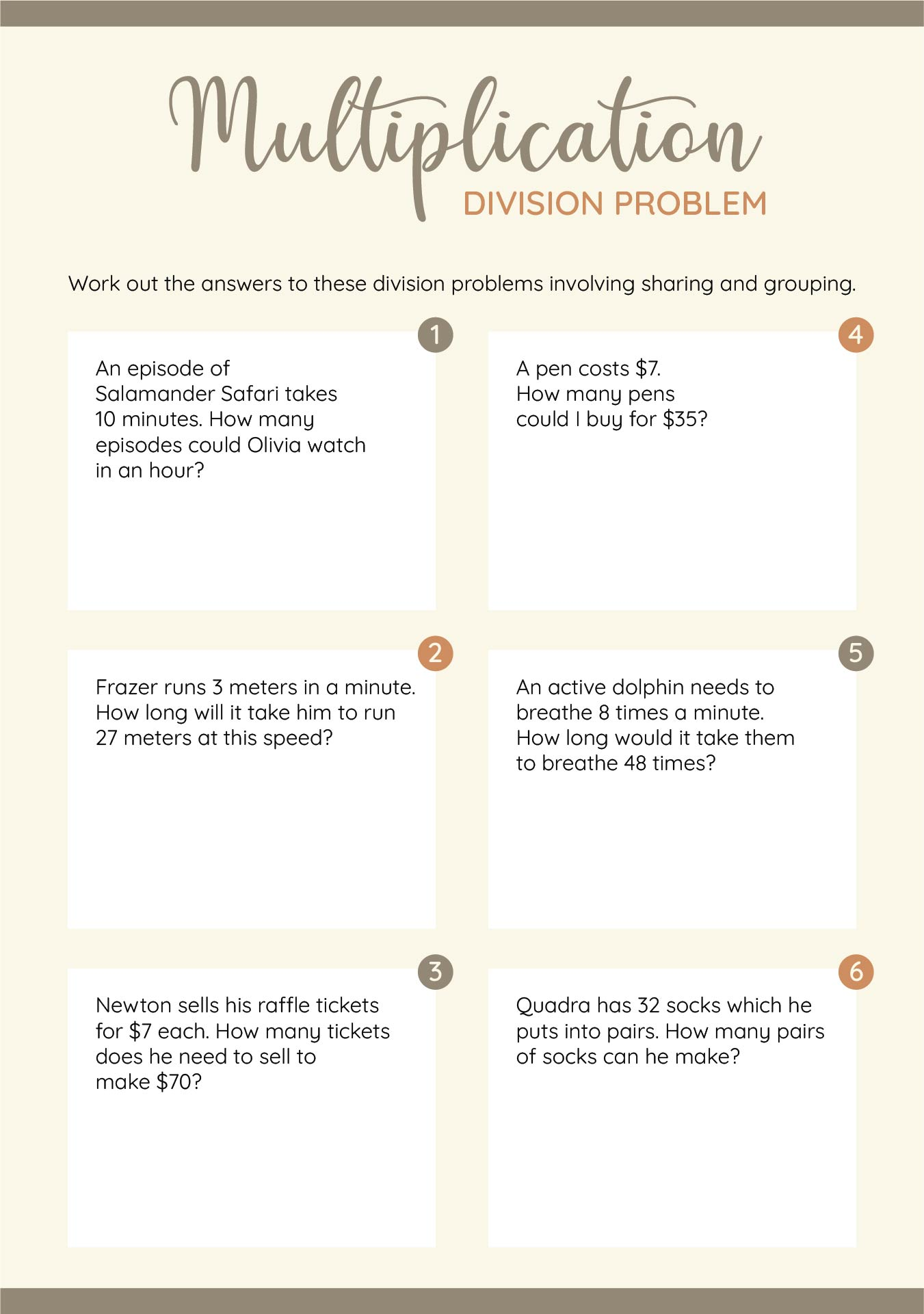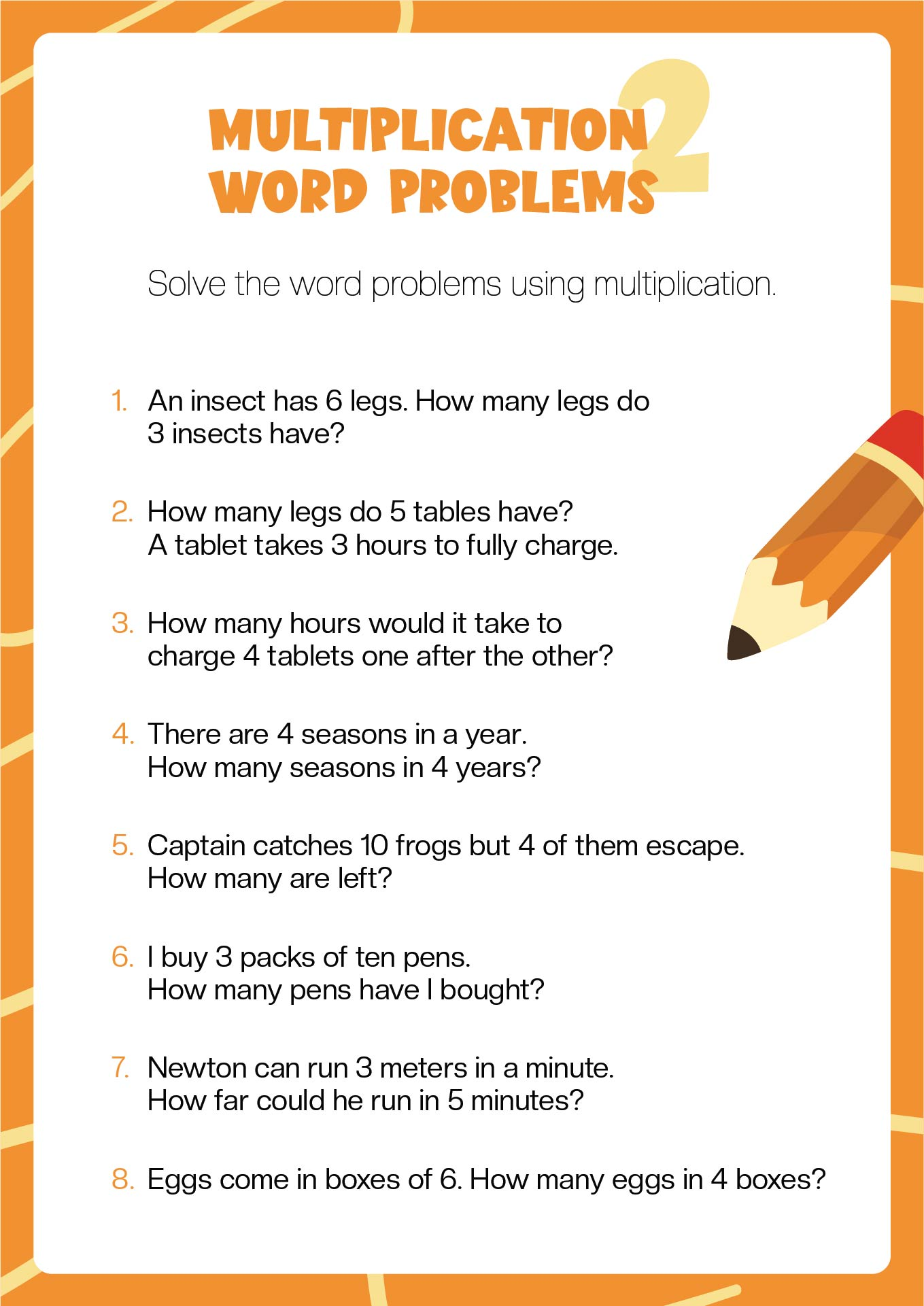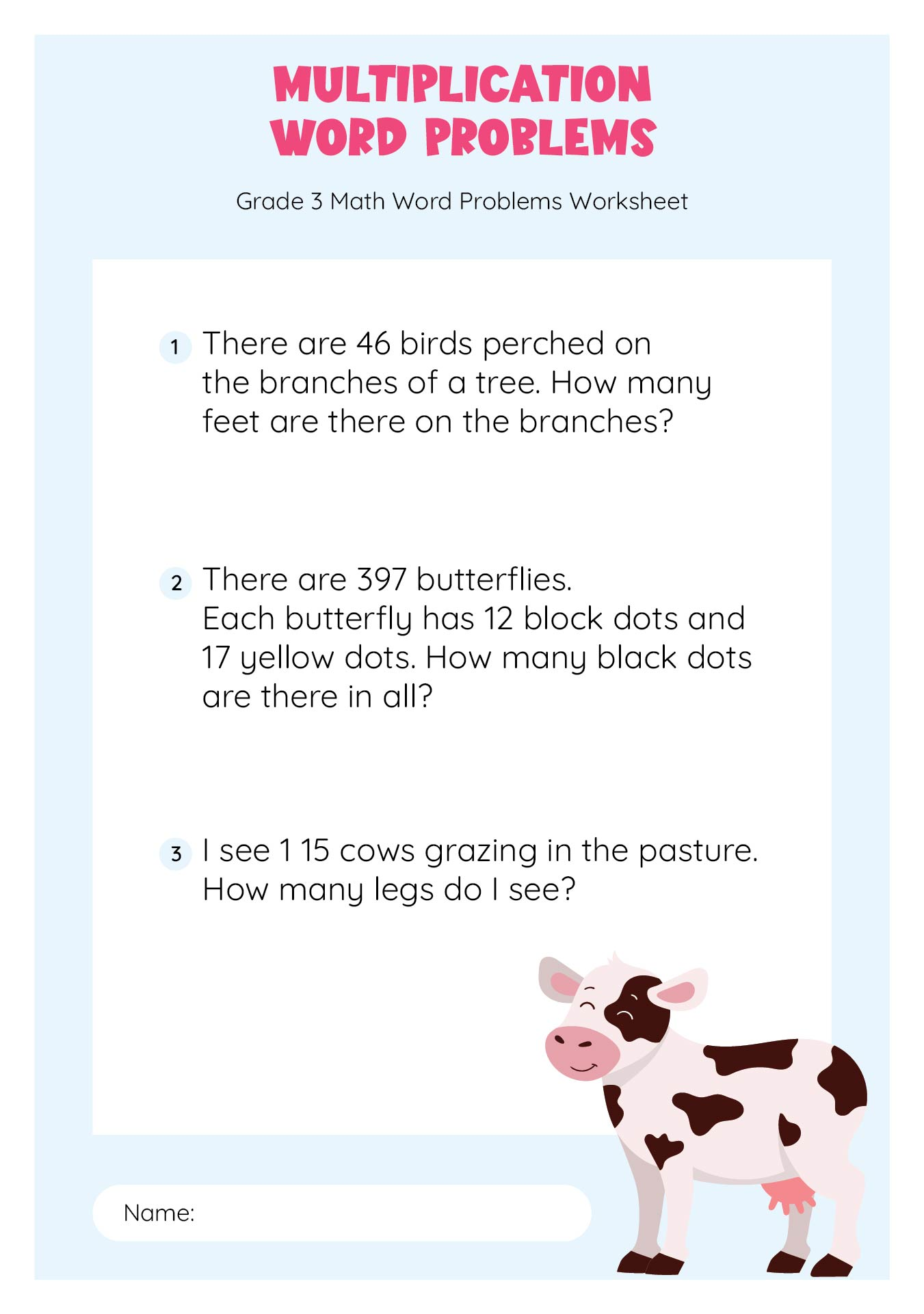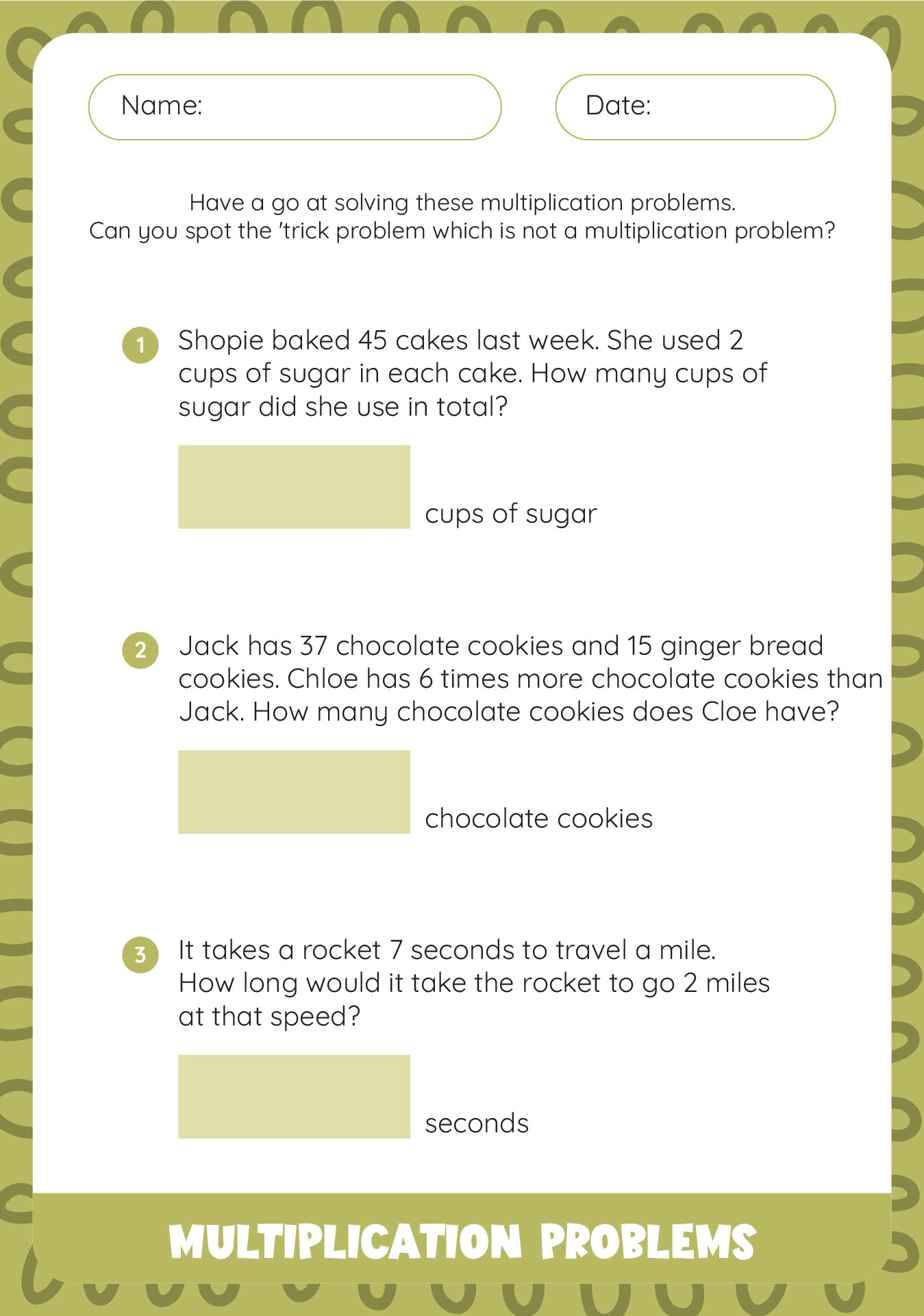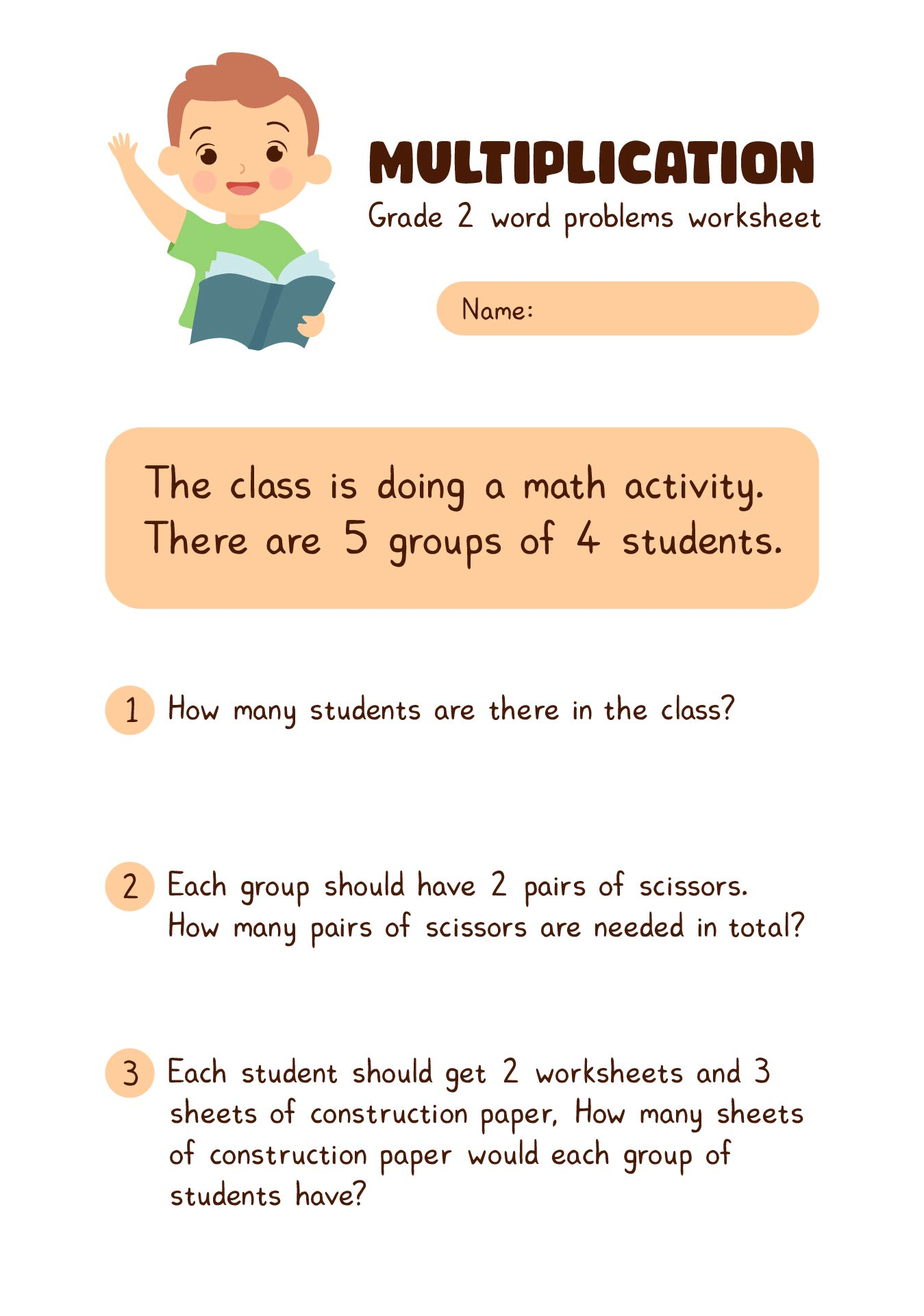## How do you teach the concept of situations and solutions in multiplying numbers?

### 1. Repeating

Memorization is not the first step in teaching youngsters how to multiply. Instead, your first priority should be to explain to them what multiplication truly entails.

Repeated addition is what multiplication is. It's that easy! You combine groups of the same quantity. You should add three groups of two cherries, like in the example below.

You don't even need to use the word "multiplication" at this point.

However, it's crucial to apply manipulatives and images. This aids children in fully grasping the idea.

For instance, you may ask kids to draw 4 ice cream cones with 2 scoops each to represent the equation "2 + 2 + 2 + 2 = 8". Alternatively, you may invite them to express another equation, such as "4 + 4 + 4 equals 12," using popsicle sticks or counting bears.

### 2. Explain the Multiplication Table

You can gradually introduce a new method for demonstrating repeated addition. You might explain that you are adding 4 groups of 3 instead of saying "5 + 5 + 5 equals 15." Then, ask kids to describe the issue in both ways:

5 + 5 + 5 + 5 = 20 and 5 x 4 = 20.

Children can also be encouraged to think of multiplication as an array. Rows and columns make up an array. Using this style makes it simple to arrange things. Children can then represent multiplication using X rows and Y beads.

It may be beneficial to keep connecting this concept to repeated addition at this stage. Therefore, the illustration above demonstrates that 2 x 6 = 12 and 6 + 6 = 12.

Children get a solid understanding of the subject by continuing to utilize visuals and demonstrating the numerous methods to communicate it.

You may also go backward to help your second-graders understand multiplication. Asking kids to organize an array into a specific number of rows or columns is preferable to giving them an array that has already been created. For instance, youngsters are required to arrange three columns of four squares each.

Kids can then write the equation as 2 x 3 = 6 or 2 + 2 + 2 = 6.

### 3. Try using different manipulatives

Maybe your kids are becoming tired of connecting blocks and Cuisenaire Rods. Why not have students make felt ice cream cones that they can fill with scoops of ice cream? Or use felt to cut out some rockets! Use a topic that your kids will find engaging to make visualizing multiplication problems entertaining.